Back to Tesla coil project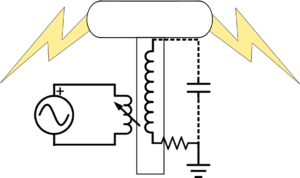I didn't really have a good intuition for how the parameters of the Tesla coil mater, so I decided to make a toy model and see how that model depends on the parameters. The model consists of two coupled circuits. The coupling of the two circuits happens through the mutual inductance of the primary and secondary winds of the transformer. Both sides of the circuit are grounded to the same high quality ground (I drew the figure wrong). This is probably not a good model for a spark gap Tesla coil since the capacitance of the driving circuit is typically large and here it is approximated as zero. The two coupled differential equations that you get from the circuit diagram shown to the right are: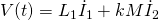and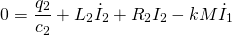whereis the mutual inductance of a perfectly coupled set of coils.  Now, assuming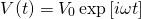allows us to write the equations like,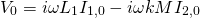and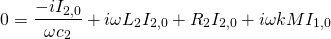.

Now, doing the algebra and we get that the impedance,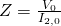, is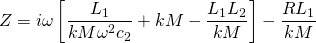From the impedance we can derive the resonant frequency,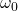, and the quality factor.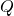. The resonant frequency we get by minimizing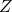with respect to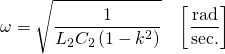or,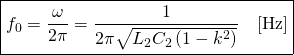So, the coupling constant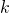will tune the resonant frequency of the Tesla Coil. As you can see in figure 1, as long as the coupling constant is relatively small, it doesn't really effect the resonant frequency. Similarly, in figure 2 you can see that as long as the coupling constant is small, the quality factor is also unchanged as a function of.